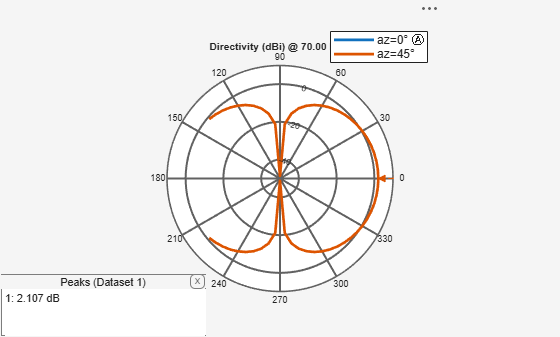# patternElevation

Elevation plane radiation pattern of antenna or array

## Syntax

``patternElevation(object,frequency,azimuth)``
``patternElevation(object,frequency,azimuth,Elevation=Value)``
``directivity = patternElevation(object,frequency,azimuth)``
``directivity = patternElevation(object,frequency,azimuth,Elevation=Value)``

## Description

example

````patternElevation(object,frequency,azimuth)` plots the 2-D radiation pattern of the antenna or array object in the elevation plane over a specified frequency. Azimuth values defaults to zero if not specified.```

example

````patternElevation(object,frequency,azimuth,Elevation=Value)` uses the specified range of elevation angles to plot the 2-D radiation pattern of the antenna or array in the elevation plane over a specified frequency. Azimuth values default to zero if not specified. Elevation values default to `[-90:1:90]` if not specified.```
````directivity = patternElevation(object,frequency,azimuth)` returns the directivity of the antenna or array object at specified frequency. Azimuth values default to zero if not specified.```
````directivity = patternElevation(object,frequency,azimuth,Elevation=Value)` uses the specified range of elevation angles to calculate the directivity of the antenna or array object over a specified frequency. Azimuth values default to zero if not specified. Elevation values default to [-90:1:90] if not specified.```

## Examples

collapse all

Calculate and plot the elevation pattern of the helix antenna at 2 GHz.

```h = helix; patternElevation (h, 2e9);```Calculate and plot the elevation radiation pattern of the dipole antenna at 70 MHz at elevation values of 0 and 45.

``` d = dipole; patternElevation(d,70e6,[0 45],Elevation=-140:5:140);```## Input Arguments

collapse all

Antenna or array from catalog, custom antenna or array, or installed antenna on a platform to calculate/plot the elevation plane directivity pattern specified as an antenna or array object.

Example: `dipole`

Example: `rectangularArray`

Frequency to calculate/plot elevation directivity pattern, specified as a scalar in Hz.

Example: 70e6

Data Types: `double`

Azimuth angle values, specified as a vector in degrees.

Example: `[0 45]`

Data Types: `double`

Range of elevation angles to calculate the directivity of antenna or array, specified as a vector in degrees.

Example: `Elevation=0:1:360`

Data Types: `double`

## Output Arguments

collapse all

Antenna or array directivity, returned as a matrix in `dBi`. The matrix size is the product of number of elevation values and number of azimuth values.

## Version History

Introduced in R2015a